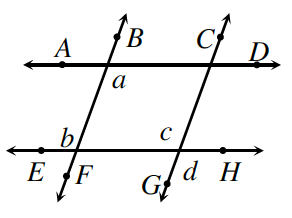### Home > INT2 > Chapter 7 > Lesson 7.1.4 > Problem7-49

7-49.

Carolina compares her proof to Penn’s work in problem 7-39. Like him, she wants to prove that if $\overline{AD} // \overline{EH}$ and $\overline{BF} // \overline{CG}$, then $a = d$. Unfortunately, her statements are in a different order. Examine her proof below and help her decide if her statements are in a logical sequence to prove that $a = d$.Statements

Reasons

1. $\overline{AD} // \overline{EH}$

Given

1. $a = b$

If lines are parallel, alternate interior angles are equal.

1. $a = c$

Substitution

1. $\overline{BF} // \overline{CG}$

Given

1. $b = c$

If lines are parallel, corresponding angles are equal.

1. $c = d$

Vertical angles are equal.

1. $a = d$

SubstitutionBe sure to start with the "Given" statements. Then, if lines are parallel, which pairs of angles will be congruent?